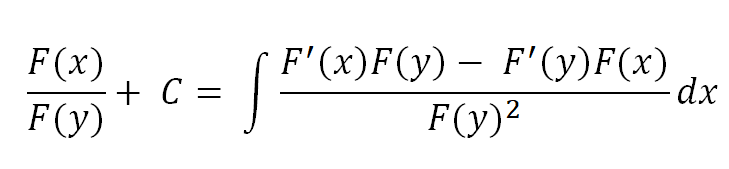# Integral or Antiderivative Quotient rule with Examples: Integration by parts Division Formula

Home  > Math Article > Integration by parts quotient rule

Page Contents

## What is Integration Quotient Rule and Its formulas?

The integral quotient rule is the way of integrating two functions given in form of numerator and denominator. This rule is also called the Antiderivative quotient or division rule.

The formula for the Integral Division rule is deduced from the Integration by Parts u/v formula.Assume a divisible function

⇒ F(x) = $\frac { F ( u ) } { F ( v ) }$

Differentiate the function F(x)

$F'( x ) = \frac { F'( u ) F( v ) – F'( v ) F( u ) } { F( v ) ^ { 2 } }$

Now integrate the F'( x ) then we get

$\frac { F ( u ) } { F ( v ) } + C = \int _ { } ^ { } \frac { F'( u ) F( v ) – F'( v ) F( u ) } { F( v ) ^ { 2 } } dx$

This formula has own limitation so not to completely rely on to integrate fraction functions.

### Point to be remembered for Integral quotient/division rule

1. This is the analogous result of integration by parts using the quotient rule.
2. The division rule is best for differentiation and the Product rule is best for integration
3. The quotient rule requires an extra integration for solving the integral problem than the integration by parts rule.
4. The Antiderivative quotient rule is another form of integration by parts formula and it has very limited use.

## How to Integrate a fraction or division function using the quotient rule?

To solve the fraction integral function you can use the chain rule which gives you substitution and the product rule which gives you integration by parts.

Using these both rules you can solve or show your final solution according to this result-From this formula, you can get the idea of what you need to further integrate the divisible function.

Or you can use the integration by parts rule to solve division integral functions by taking one function as u and the other as v according to the ILATE rule.

I = Inverse trigonometric function

L = Logarithmic function

A = Algebraic function

T = Trigonometric function

E = Exponential Function

Integration by parts u/v form-

$\int _ { } ^ { } udv = u.v – \int _ { } ^ { } v.du$

Note: There is no direct rule which solve the fraction or division integral problem as product rule does.

We use different-different methods to cope with this type of problem like Integration Partial fraction for repeated or non repeated linear factor, Integration Substitution Method, Integration by parts, etc.

### Indefinite Integration of a quotient polynomial using substitution examples

Example 1: $\int _ { } ^ { } \frac { z + 5 } { z ^ { 2 } + 10z } dz$

Solution-

⇒ u = z² + 10z

⇒ du = (2z + 5)dz

Divide by 2, L.H.S and R.H.S

$\frac { du } { 2 } = \frac { 2 ( z + 5 ) dz } { 2 }$

$\frac { du } { 2 } = ( z + 5 ) dz$

Put all vaules in problem

= $\frac { 1 } { 2 } \int _ { } ^ { } \frac { du } { u }$

= $\frac { 1 } { 2 } ln|u| + C$

= $\frac { 1 } { 2 } ln|z ^ { 2 } + 10z | + C$  Answer

Example 2: $\int _ { } ^ { } \frac { 3z + 7 } { z ^ { 2 } + 4 } dz$

Solution-

Separate the function in such way we get same denominator after it

= $3 \int _ { } ^ { } \frac { z } { z ^ { 2 } + 4 } dz + 7 \int _ { } ^ { } \frac { 1 } { z ^ { 2 } + 4 } dz$

Now substitution:

⇒ u = z² + 4

⇒ du = 2z dz

Take this function $\int _ { } ^ { } \frac { z } { z ^ { 2 } + 4 } dz$

and substitute the above value in given function

= $\int _ { } ^ { } \frac { \frac { 1 } { 2 } du } { u }$ = 1/2 ln|u| + C

= 1/2 ln (z² + 4) + C  Answer

## FAQ

### What is the use of the Integral quotient rule?

The integral quotient rule is mostly used to determine what you need to find the integration of the two-division functions. The quotient rule is originated from differentiation and the integral divisible formula is just another form of integration by parts to further check how to integrate divisible functions

### What are the Anti product division rule and their use?

Anti-product division is the other name of the integral divisible rule and it works best for differentiation not for integration.

### How to solve Fraction or Division integration quickly?

There is no short cut to solve integral divisible function quickly. But you can take an idea from the Antiderivative quotient rule or use other methods like Integral substitution, Integration partial fraction, or Integration by parts.
##### Subscribe to Blog via Email

Join 2 other subscribers

Share it...